##CS 660: Combinatorial Algorithms AVL TREES

[To Lecture Notes Index]
San Diego State University -- This page last updated October 27, 1995## Height-Balanced Trees

Height of a tree is the length of the longest path from the root to a leaf.

A binary search tree T is a height-balanced k-tree or HB[k]-tree, if each node in the tree has the HB[k] property

A node has the HB[k] property if the height of the left and right subtrees of the node differ in height by at most k.

A HB tree is called an AVL tree.

Adel'son-Vel'skii and Landis first defined HB trees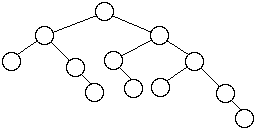Worst Case AVL TreesLet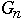= the number of nodes in worst case AVL tree of height n

We have: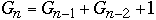Fibonacci Numbers,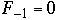,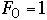n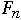0 1 1 1 2 1 2 4 2 3 7 3 4 12 5 5 20 8 6 33 13

We get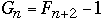Butwhere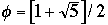So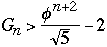Now take any AVL tree of height h with M nodes, we have

M >=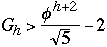So M + 2 >h < 1..4404 lg(M + 2) - 0.328

Theorem ( Adel'son-Vel'skii and Landis 1962) The height of a balanced tree with N internal nodes always lies between lg (N +1 ) and 1..4404 lg(M + 2) - 0.328

### Insertion into a AVL Tree

Case 1
Add node to subtree 3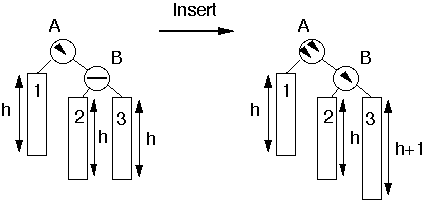Perform Single Rotation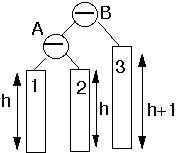### Insertion into a AVL Tree

Case 2
Insert into subtree 2 or 3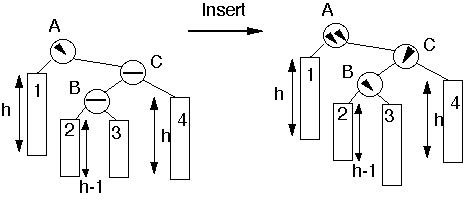Double Rotation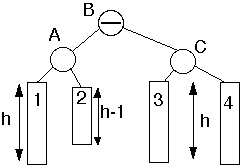### Performance

Theorem. An insertion into an AVL tree requires at most one rotation to rebalance a tree. A deletion may require lg(N) rotations to rebalance the tree.

Simulation Results
 n C(n) E[ h(n) ] R(n) 5 2.2 3.0 0.213 10 2.907 4.0 0.318 50 4.930 6.947 0.427 100 5.889 7.999 0.444 500 8.192 10.923 0.461 1000 9.202 11.998 0.463 5000 11.555 14.936 0.465 10000 12.568 15.996 0.465
n = number of nodes in the tree

C(n) = average number of comparisons to find a key

E[ h(n) ] = expected height of tree

R(n) = average number of rotations per insertion/deletion